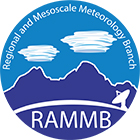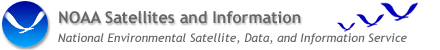## Effect of bit depth (8-bit vs. 10-bit) on the noise level of GOES images and image products

This section was generated to assess the effect of 8-bit vs. 10-bit data precision on the quality of GOES imagery and image products generated from those images. Both 2-byte (10-bit) imagery and the same imagery reduced to 1-byte (8-bit) precision were analyzed to determine the effect of bit depth on noise levels. This analysis was accomplished using spatial structure analysis to determine the noise between adjacent pixels in imagery from real earth scenes.

### Effect of bit depth on GOES images

In the figures below GOES IR bands 4, 5, and 2 are analyzed in both 2-byte (10-bit) and 1-byte (8-bit) mode for noise in temperature units. Each figure gives two determinations of the noise level (the two vertical shaded bars for each band) using spatial structure analysis. (The third, longer bar is a measure of the standard deviation of the measurements in the portion of the GOES images that were analyzed. This value needs to be near the other two noise numbers to indicate that the analysis was performed on an area of the image where there is little spatial variability.)

In the top figure are the noise levels for 10-bit data, and in the bottom figure are the noise levels for 8-bit data. Because of the loss of precision due to the conversion from 10-bits to 8-bits, there is a resulting increase in existing noise from the top to the bottom figure. For both GOES band-4 and 5 the noise is increased from about 0.30 K to about 0.33 K [for a 288 K scene temperature], or an increase of about 10% over the 10-bit value, but for GOES band-2 the noise is only slightly, if at all, increased (from about 0.44 K to about 0.45 K). The reason for the nil effect on GOES band-2 is due to the fact that the noise level of band-2 data for most earth scenes is already larger than the limiting precision of 8-bit data. This was explained in the previous section which can be reached from the return link at the bottom of the page. This situation is different for both GOES band-4 and 5, where 8-bit data limits the precision of data in those bands.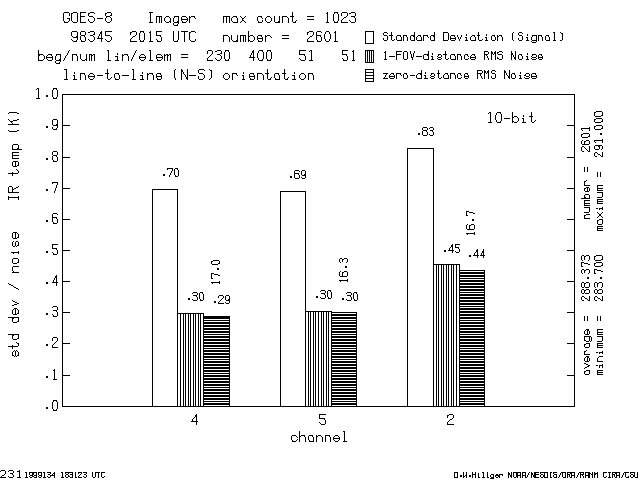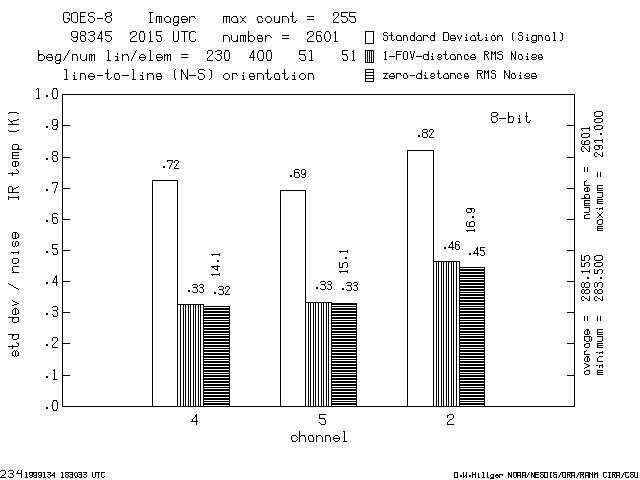Another indication of the effect of the increased noise in the figures above are the signal-to-noise numbers written vertically above the noise bars mentioned above. For GOES band-4 and 5 the signal-to-noise decreases from about 16-17 to about 14-15, a decrease of more than 10%.

### Effect of bit depth on GOES image products

The effect of bit depth on GOES imagery is not only manifest in the band images, but in image products generated from those images. The figures below show the effect of bit depth on the noise level of the GOES fog product (a band-4 minus band-2 difference) and the effect of bit depth on another band-difference product generated from GOES band-4 and 5.

In the figure below, the shaded bars indicate the noise levels determined for the GOES fog product. The first set of bars are the noise levels for the fog product generated from 10-bit data, and the second set of bars are the noise levels for the fog product generated from same data but reduced to 8-bit precision. [In both cases the fog product is saved as an 8-bit image, where 1 count represents a temperature difference of 0.1 K. This is enough precision so that no additional noise is introduced into the fog product by storing the image at this bit depth. The only limit on precision of the fog product is due to the bit depth of the original data.] However, the noise has increased from about 4.0 counts [0.40 K] for the product generated from 10-bit data to about 4.4 counts [0.44 K] for the product generated from 8-bit data. This is an increase of about 10% in noise, similar to the increase in existing noise for GOES band-4 alone.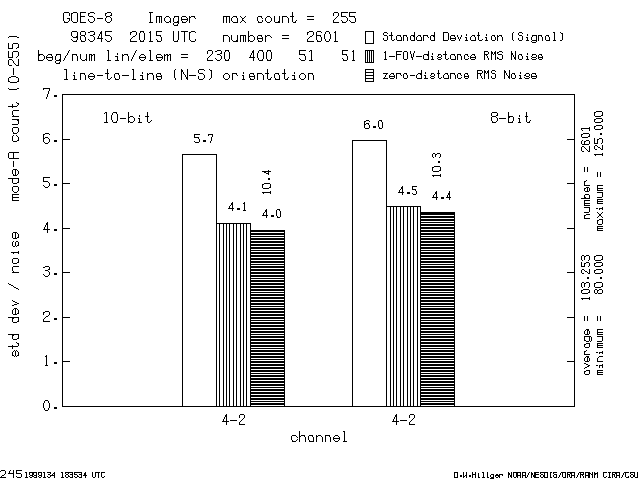In the figure below, the shaded bars indicate the noise levels determined for a band-4 minus band-5 difference product. The first set of bars are the noise levels for this product generated from 10-bit data, and the second set of bars are the noise levels for the same band-difference product generated from 8-bit data. [Again, in both cases the band-difference product is saved as an 8-bit image, where 2 count represents a temperature difference of 0.1 K. This is enough precision so that no additional noise is introduced into the band-difference product by storing the image at this bit depth. The only limit on precision of the fog product is due to the bit depth of the original data.] However, the noise has increased from about 12 counts [0.6 K] for the product generated from 10-bit data to about 13 counts [0.65 K] for the product generated from 8-bit data. This is an increase of about 10% in noise, similar to that for the GOES fog product.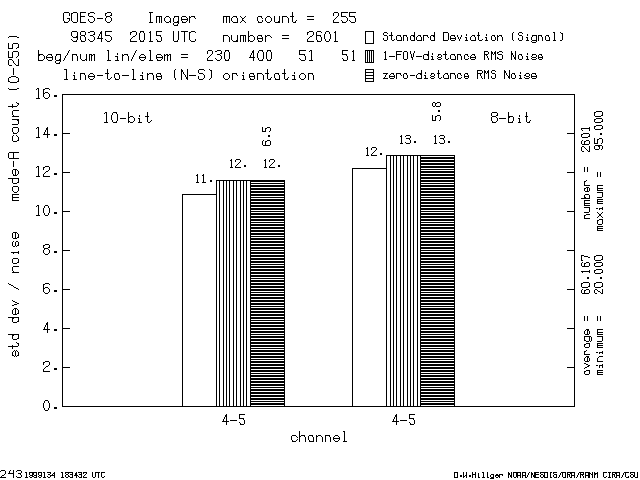### Summary

The effect of bit depth on the quality of GOES images and image products generated from GOES band images has been determined for one sample of data. The reduction from 10-bit to 8-bit precision results in an increase in existing noise by about 10% for GOES band-4 and 5. This effect is similar for both these individual GOES bands and for two image products generated from GOES data.

• This Web page maintained by Don Hillger, PhD
• Web site courtesy of CIRA/Colorado State University.